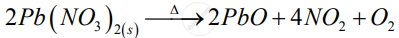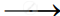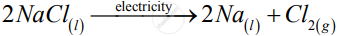# Decomposition Reactions Require Energy Either in the Form of Heat Or Light Or Electricity for Breaking Down the Reactants. Write One Equation Each for Decomposition Reactions Where Energy is Supplied in the Form of Heat, Light and Electricity - Science

Decomposition reactions require energy either in the form of heat or light or electricity for breaking down the reactants. Write one equation each for decomposition reactions where energy is supplied in the form of heat, light and electricity

#### Solution

Those reactions in which a compound splits up into two or more simpler substances are known as decomposition reactions.

1)2)

2AgCl_((s)) + Sunlight2Ag_(s) + Cl_(2(g))

3)Concept: Types of Chemical Change or Chemical Reaction - Decomposition Reactions
Is there an error in this question or solution?